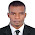## Wednesday, August 8, 2018

Engineers, site managers, and quantity surveyors are always faced with the challenge of specifying as accurately as possible, the quantity of materials needed to execute a specific item of work. In this post, we are going to explain how you can estimate the quantity of mortar (cement and sand) needed to lay blocks per square metre of wall.

It is well known that we need about 10 blocks to build one square metre of wall.

Simple Proof;
Area of wall = 1 m2
Planar area of one standard block (Nigeria) = 450mm x 225mm = 0.101 m2
Therefore, number of block required (disregarding mortar) = 1/0.101 = 9.99 (say 10 blocks)

Now, how do we estimate the quantity of mortar needed to lay the blocks? First of all, let us look at the dimensions of 9 inches block with hole (most popular block for building in Nigeria).

From the image above, we can say that the cross-sectional area of the block that receive horizontal mortar is;

Ab = (0.45 x 0.225) - 2(0.15 x 0.125) = 0.0637 m2

Now, let us assume that the mortar will be 25 mm thick

The typical arrangement of blocks in a one square metre wall is given below;

Therefore, we can estimate the volume of mortar as such;

Vertical mortar = 8 x (0.025 x 0.225 x 0.275) = 0.0123 m3
Horizontal mortar = 10 x (0.0637 x 0.025) = 0.0159 m3
Total = 0.0282 m3

Therefore the volume of mortar required to lay one square metre of 9 inches block (with hole) can be taken as 0.03m3 for all practical purposes.

To go further, let us assume that we have 150 m2 of wall, and we want to estimate the quantity of cement and sand for mortar needed to lay the blocks.

The volume of mortar required = 0.03 x 150 = 4.5 m3

Typical mix ratio for mortar (laying of blocks) is 1:6

Cement
Quantity of cement required = 1/7 x 1440 kg/m3 = 205.71 kg
Making allowance for shrinkage between fresh and wet concrete = 1.54 x 205.71 = 316.79 kg
Quantity of cement required in bags = 316.79/50 = 6.33 bags

For 4.5m3;
Provide = 4.5 x 6.33 = 29 bags of cement + allowance for waste as appropriate.

Sand
Quantity of sand required = 6/7 x 1600 kg/m3 = 1371.428 kg
Making allowance for shrinkage between fresh and wet concrete = 1.54 x 1371.428 = 2112 kg

For 4.5m3;
Provide = 4.5 x 2112 = 9504 kg of sharp sand + allowance for waste as appropriate.

So you can order 10 tonnes of sharp sand and 32 bags of cement for this job.

Thank you for visiting Structville today, and God bless.

1.Nice post.
Dry volume = 1.54 x Wet volume for concrete
Dry volume = 1.35 x Wet volume for mortar please confirm a lot of arguments on this

2.This is an awesome one bro.
It makes sense but truth still remains that no one will accept that on site.

3.No client will accept this, this is one of the problems of Engineering in Nigeria. Your estimation is according to standard, but that's only for Company Jobs where a workman is entitled to just 70 blocks per day.

No common man will accept this, before you know it, one person that doesn't even know standard will come and quote nonsense.

Kudos bro

4.